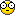# Two Sorted Array, merge them##Two Sorted Array, merge them

public int[] Merge(int[] a1, int[] a2){
int len1=a1.length()-1;
int len2=a2.length()-1;
int len=len1+len2+1;
int[] arr=new int[len];
while(len1>=0&&len2>=0){
if(a1[len1]>a2[len2])
arr[len--]=a1[len1--];
else
arr[len--]=a1[len2--];
}
if(a1.length()>a2.length()){
while(len1>0){
arr[len--]=a1[len1--];
}
}
else{
while(len2>0){
arr[len--]=a2[len2--];
}
}
return arr;
}

##Re: Two Sorted Array, merge them

Why you put this topic here?

Admin wrote:public int[] Merge(int[] a1, int[] a2){
int len1=a1.length()-1;
int len2=a2.length()-1;
int len=len1+len2+1;
int[] arr=new int[len];
while(len1>=0&&len2>=0){
if(a1[len1]>a2[len2])
arr[len--]=a1[len1--];
else
arr[len--]=a1[len2--];
}
if(a1.length()>a2.length()){
while(len1>0){
arr[len--]=a1[len1--];
}
}
else{
while(len2>0){
arr[len--]=a2[len2--];
}
}
return arr;
}

##Re: Two Sorted Array, merge them

The code is to merge two sorted array. Anything wrong here?##Re: Two Sorted Array, merge them

I mean most questions in this folder are knowledge-based questions. Then there's one algorithm question, looks strange.

Anyway, I think there should be some folders for algorithm, I have "Sorting" (which you already have), "recursive", "dynamic programming", "bit operation", "binary search", "random algorithm" and "other algorithm" on my computer. I hope you could also create these forums.

##Re: Two Sorted Array, merge them

I already created some topics as what you listed. By the way, what is random algorithm?

viterbi wrote:I mean most questions in this folder are knowledge-based questions. Then there's one algorithm question, looks strange.

Anyway, I think there should be some folders for algorithm, I have "Sorting" (which you already have), "recursive", "dynamic programming", "bit operation", "binary search", "random algorithm" and "other algorithm" on my computer. I hope you could also create these forums.

##Re: Two Sorted Array, merge them

Random algorithm is the name I gaveIt includes the algorithms that deal with randomness, which includes the classic algorithms: Fisher-Yates shuffle, reservoir sampling, and some others.

Admin wrote:I already created some topics as what you listed. By the way, what is random algorithm?

viterbi wrote:I mean most questions in this folder are knowledge-based questions. Then there's one algorithm question, looks strange.

Anyway, I think there should be some folders for algorithm, I have "Sorting" (which you already have), "recursive", "dynamic programming", "bit operation", "binary search", "random algorithm" and "other algorithm" on my computer. I hope you could also create these forums.

##Re: Two Sorted Array, merge them

Sponsored contentPermissions in this forum:
You cannot reply to topics in this forum